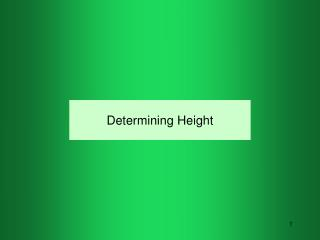DownloadDownload PresentationDetermining Height

# Determining Height

Télécharger la présentation## Determining Height

- - - - - - - - - - - - - - - - - - - - - - - - - - - E N D - - - - - - - - - - - - - - - - - - - - - - - - - - -
##### Presentation Transcript

1. Determining Height

2. Introduction • For many landscape jobs it is important to be able to determine the height of features in the landscape. • Trees • Buildings • Etc. • The best equipment and method to use is determined by the desired accuracy and precision of the data. • The equipment and methods used can be divided into two categories: • Estimates • Measurements.

3. Estimate Methods • Shadow • Line of sight • Felling • Fixed angle

4. Estimating HeightShadow Method • The shadow length of all objects is proportional to their height. • The height of an object can be determined by measuring the shadow length of an object with a known height and comparing it to the length of the shadow for the unknown height.

5. HeightShadow Method-Example Determine the height of the tree.

6. HeightShadow Method Advantages • No surveying equipment • Easy math • Disadvantages • Requires sunny day • Must have clear space to see shadows. • Low precision

7. HeightLine of Sight Method • The line of sight method is base on the principles of right triangles. • The ratio of the lengths of the sides of a right triangle are the same as long as the angle is the same. If two lengths of a small triangle and one side of the large triangle are known the length of the other side of the large triangle can be calculated using a ratio.

8. HeightLine of Sight--cont. • Select a stick of known height. • Move away from tree some distance and place stick in ground. • Insure it is plumb • Lay on ground and sight across top of stick to the top of the object. • Move towards or away from the stick until the sight line is aligned with the top of the stick and the top of the object. Measure the distance from the stick to your eye position. Measure the distance from your eye position to the base of the tree.

9. HeightLine of Sight Method--Example • Determine the height of the tower. • The stake and sight position form one triangle, the tower and sight position form a second triangle. • Both triangles have the same angle. • Therefore:

10. HeightLine of Sight Method--cont. Advantages: • Low tech • Doesn’t require sunny day • Adaptable to many different objects • Easy math • Disadvantages: • Difficulty establishing line of sight accurately. • Low precision • Precision is reduced if stake is not at same elevation as base of the object.

11. HeightFelling Method • The felling method estimates the height of an object by measuring the relative height with your hand and a pencil, or similar object, and then rotating the hand to lay out the same distance on the ground. • The end of the pencil is marked on the ground and the distance is measured. • Works best with a helper.

12. HeightFelling Method-Example Determine the height of the house.

13. HeightFelling Method-Example cont. Reproduce the height of the house with a pen or pencil.

14. HeightFelling Method-Example cont. Rotate the pencil 90 degrees and align your thumb with the edge of the building. Stake the end of the pencil in line with the building.

15. HeightFelling Method-Example cont. The distance from the house to the stake is the height of the house.

16. HeightFixed Angle Method • The fixed angle method uses a principle of triangles--the legs of a 45 degree triangle are the same length. • Easy way to get a 45 angle is to fold a piece of paper. • The height is determined by sighting along the hypotenuse of the triangle until the line of sight aligns with the top of the the object. • The height of the object is the distance from the object plus the eye height. The paper must held horizontal for acceptable results.

17. HeightFixed Angle Method-Example Determine the height of the tree.

18. Measuring Methods • Transit • Others

19. HeightMeasuring-Transit • With a transit the vertical angle to the top of the object can be measured using the tangent trig function. • Knowing the angle, the height of the instrument and the distance from the transit to the object, the height can be calculated.

20. HeightMeasuring-Transit Example

21. Questions